CBSE Class 12 Sample Paper for 2022 Boards (MCQ Based - for Term 1)

Class 12
Solutions of Sample Papers and Past Year Papers - for Class 12 Boards

## (c) 75 √3 cm 2     (d) 5 cm 2

This question is inspired from Example 37 - Chapter 6 Class 12   - Application of Derivatives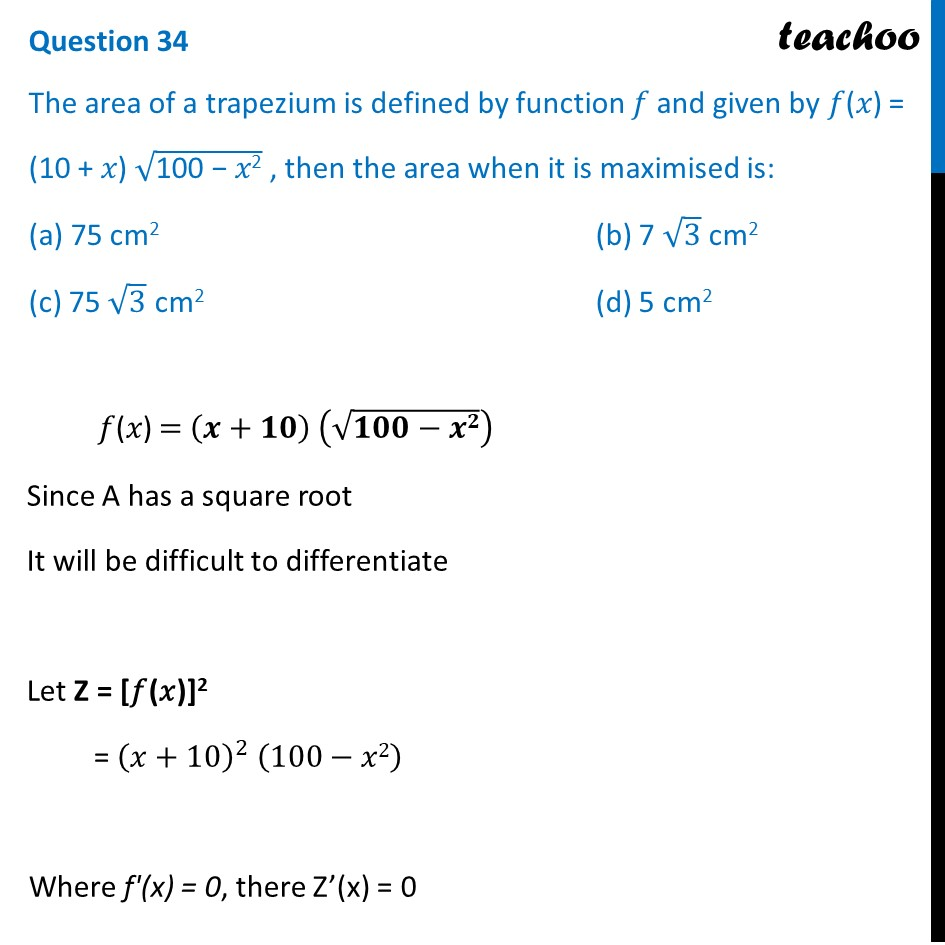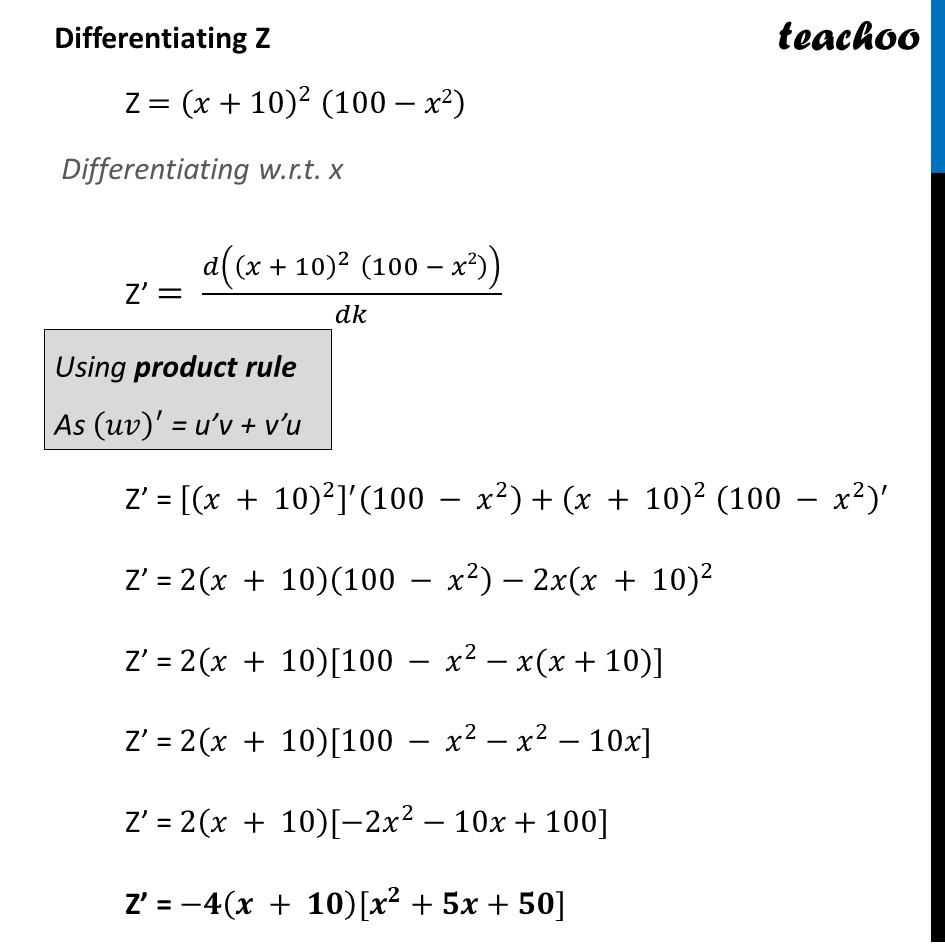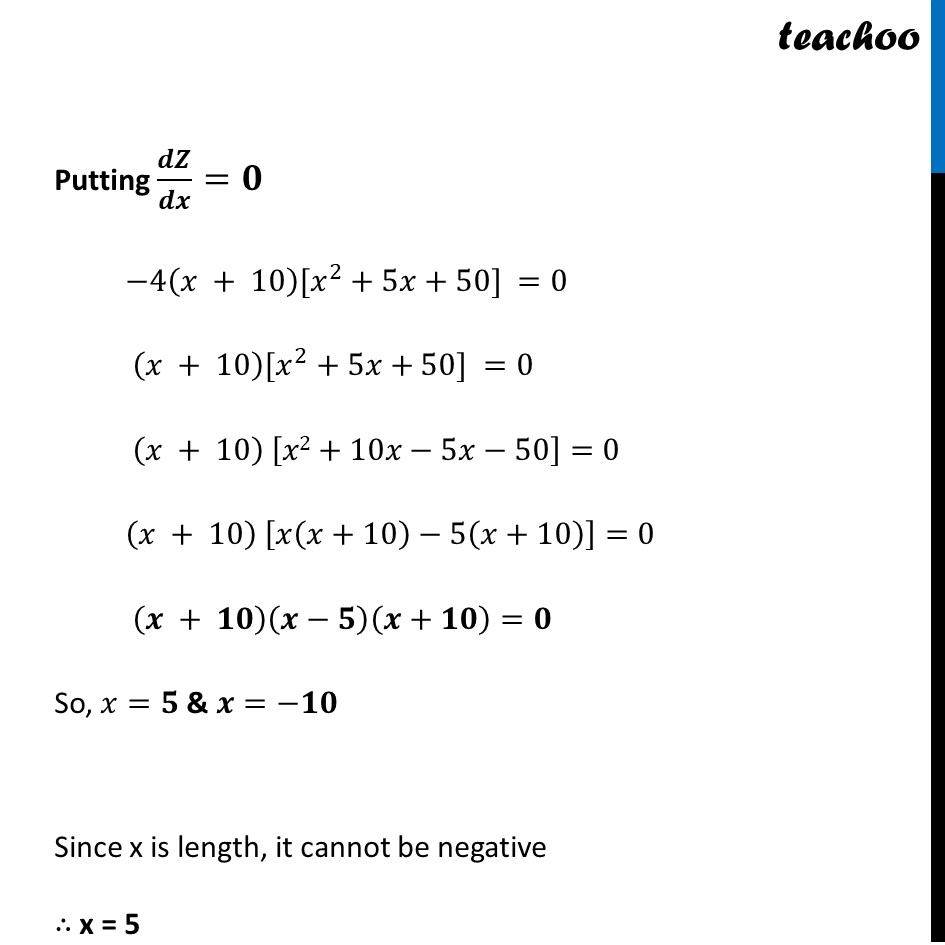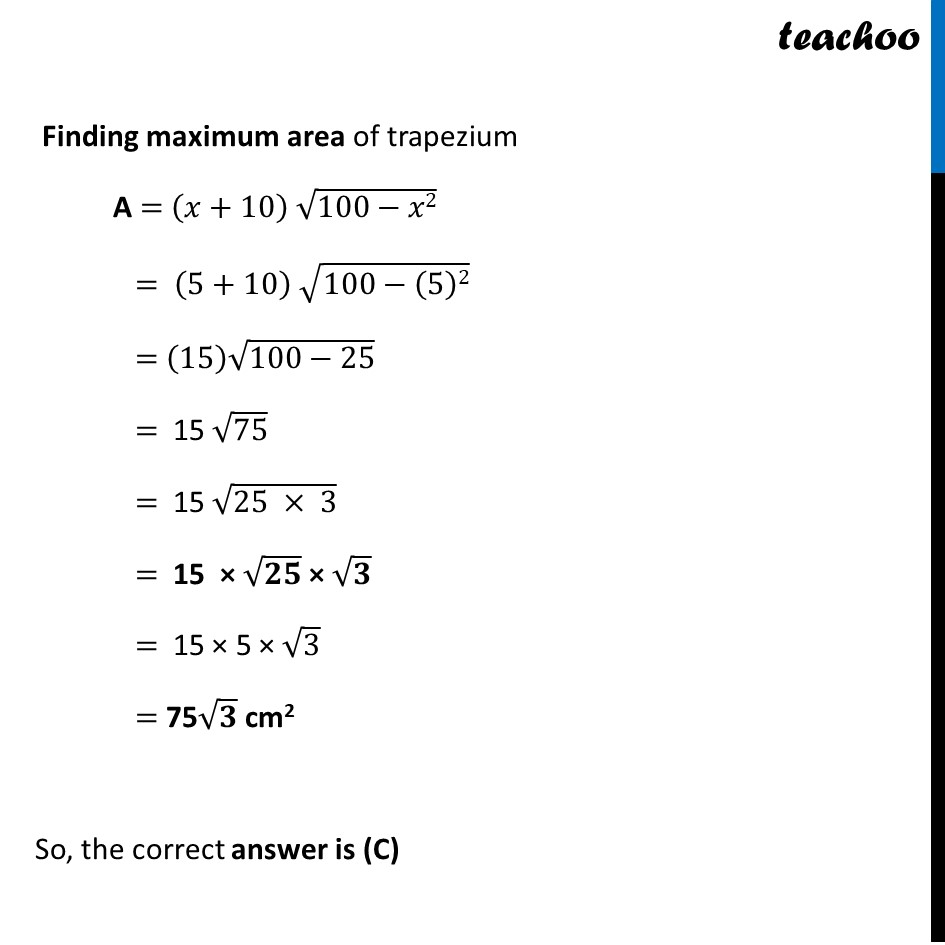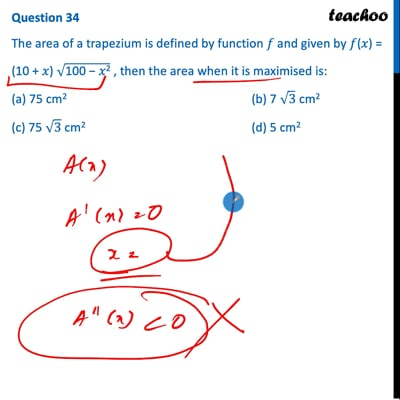This video is only available for Teachoo black users

Get live Maths 1-on-1 Classs - Class 6 to 12

### Transcript

Question 34 The area of a trapezium is defined by function 𝑓 and given by 𝑓(𝑥) = (10 + 𝑥) √("100 − 𝑥2" ) , then the area when it is maximised is: (a) 75 cm2 (b) 7 √3 cm2 (c) 75 √3 cm2 (d) 5 cm2 𝑓(𝑥) = (𝒙+𝟏𝟎) (√(𝟏𝟎𝟎−𝒙𝟐)) Since A has a square root It will be difficult to differentiate Let Z = [𝑓(𝑥)]2 = (𝑥+10)^2 (100−𝑥2) Where f'(x) = 0, there Z’(x) = 0 Differentiating Z Z =(𝑥+10)^2 " " (100−𝑥2) Differentiating w.r.t. x Z’ = 𝑑((𝑥 + 10)^2 " " (100 − 𝑥2))/𝑑𝑘 Z’ = [(𝑥 + 10)^2 ]^′ (100 − 𝑥^2 )+(𝑥 + 10)^2 " " (100 − 𝑥^2 )^′ Z’ = 2(𝑥 + 10)(100 − 𝑥^2 )−2𝑥(𝑥 + 10)^2 Z’ = 2(𝑥 + 10)[100 − 𝑥^2−𝑥(𝑥+10)] Z’ = 2(𝑥 + 10)[100 − 𝑥^2−𝑥^2−10𝑥] Z’ = 2(𝑥 + 10)[−2𝑥^2−10𝑥+100] Z’ = −𝟒(𝒙 + 𝟏𝟎)[𝒙^𝟐+𝟓𝒙+𝟓𝟎] Putting 𝒅𝒁/𝒅𝒙=𝟎 −4(𝑥 + 10)[𝑥^2+5𝑥+50] =0 (𝑥 + 10)[𝑥^2+5𝑥+50] =0 (𝑥 + 10) [𝑥2+10𝑥−5𝑥−50]=0 (𝑥 + 10) [𝑥(𝑥+10)−5(𝑥+10)]=0 (𝒙 + 𝟏𝟎)(𝒙−𝟓)(𝒙+𝟏𝟎)=𝟎 So, 𝑥=𝟓 & 𝒙=−𝟏𝟎 Since x is length, it cannot be negative ∴ x = 5 Finding maximum area of trapezium A = (𝑥+10) √(100−𝑥2) = (5+10) √(100−(5)2) = (15) √(100−25) = 15 √75 = 15 √(25 × 3) = 15 × √𝟐𝟓 × √𝟑 = 15 × 5 × √3 = 75√𝟑 cm2 So, the correct answer is (C)